# RS Aggarwal Solutions for Class 7 Maths Exercise 20B Chapter 20 Mensuration

RS Aggarwal Solutions for Class 7 Maths Exercise 20B Chapter 20 Mensuration, This exercise of RS Aggarwal Solutions for Class 7 Chapter 20 contains questions related to area between two rectangles. Students can download the PDF that is available here. Our expert specialist has prepared the RS Aggarwal Solutions for Class 7 Maths Chapter 20 Mensuration Exercise 20B to assist students in a better way, with the help of solutions provided here.

## Download the PDF of RS Aggarwal Solutions For Class 7 Maths Chapter 20 Mensuration – Exercise 20B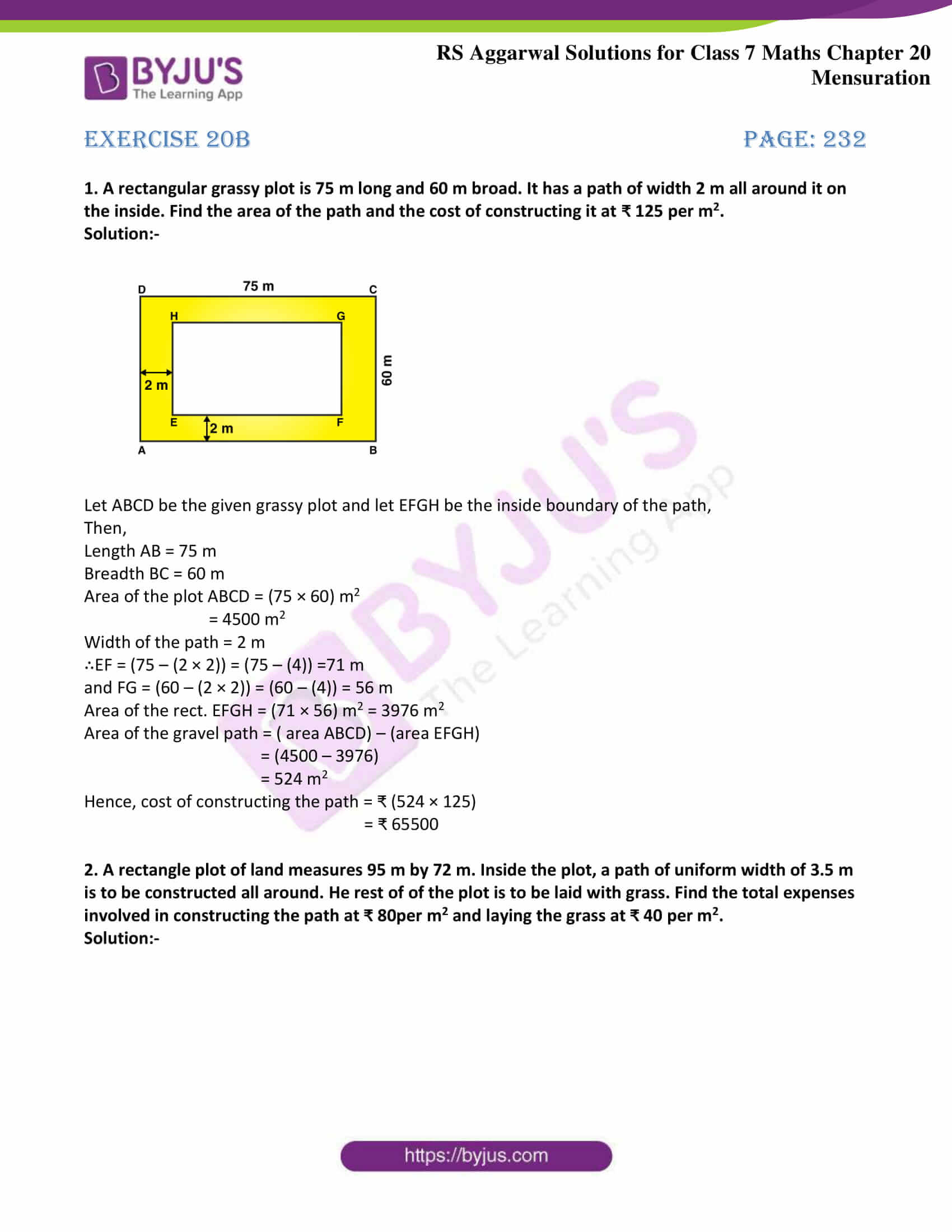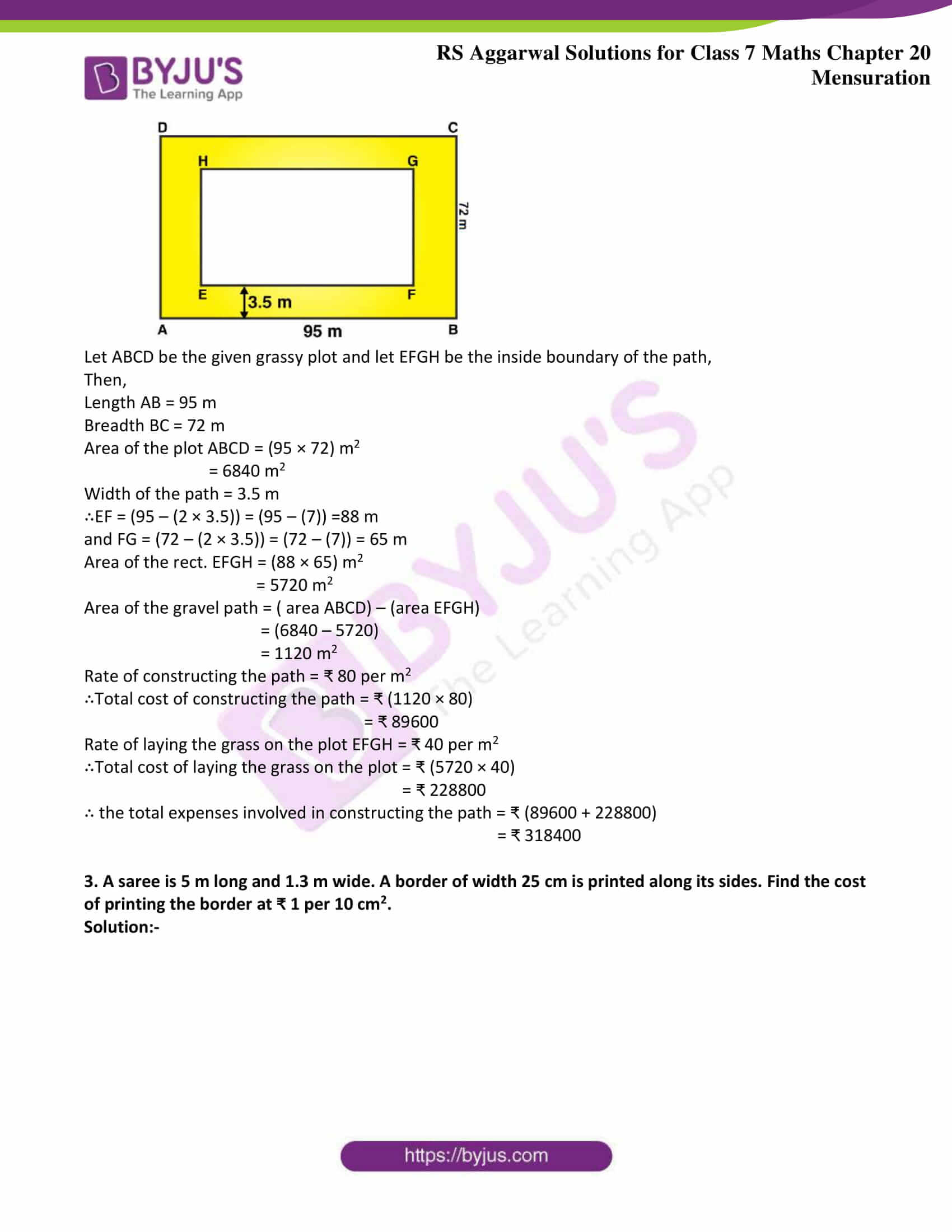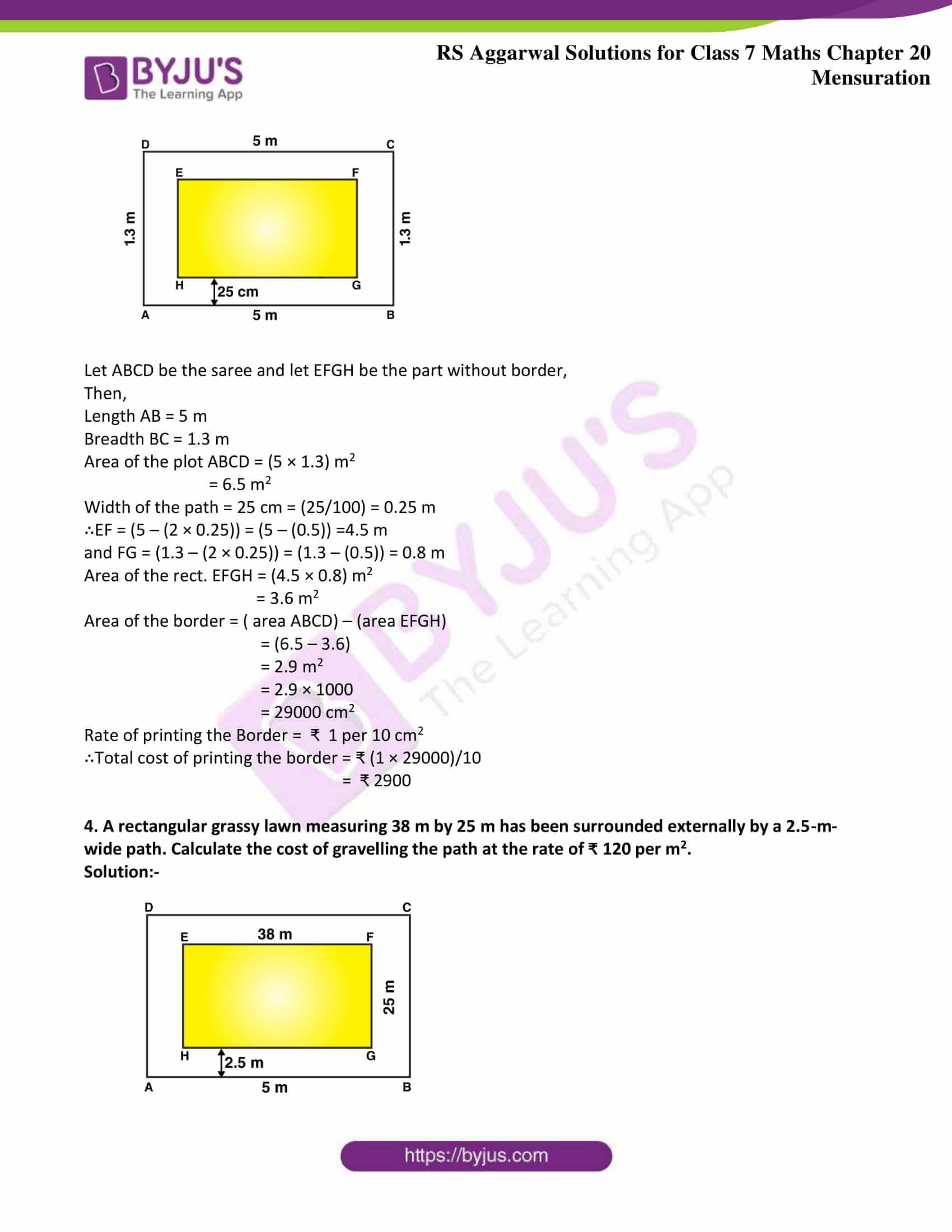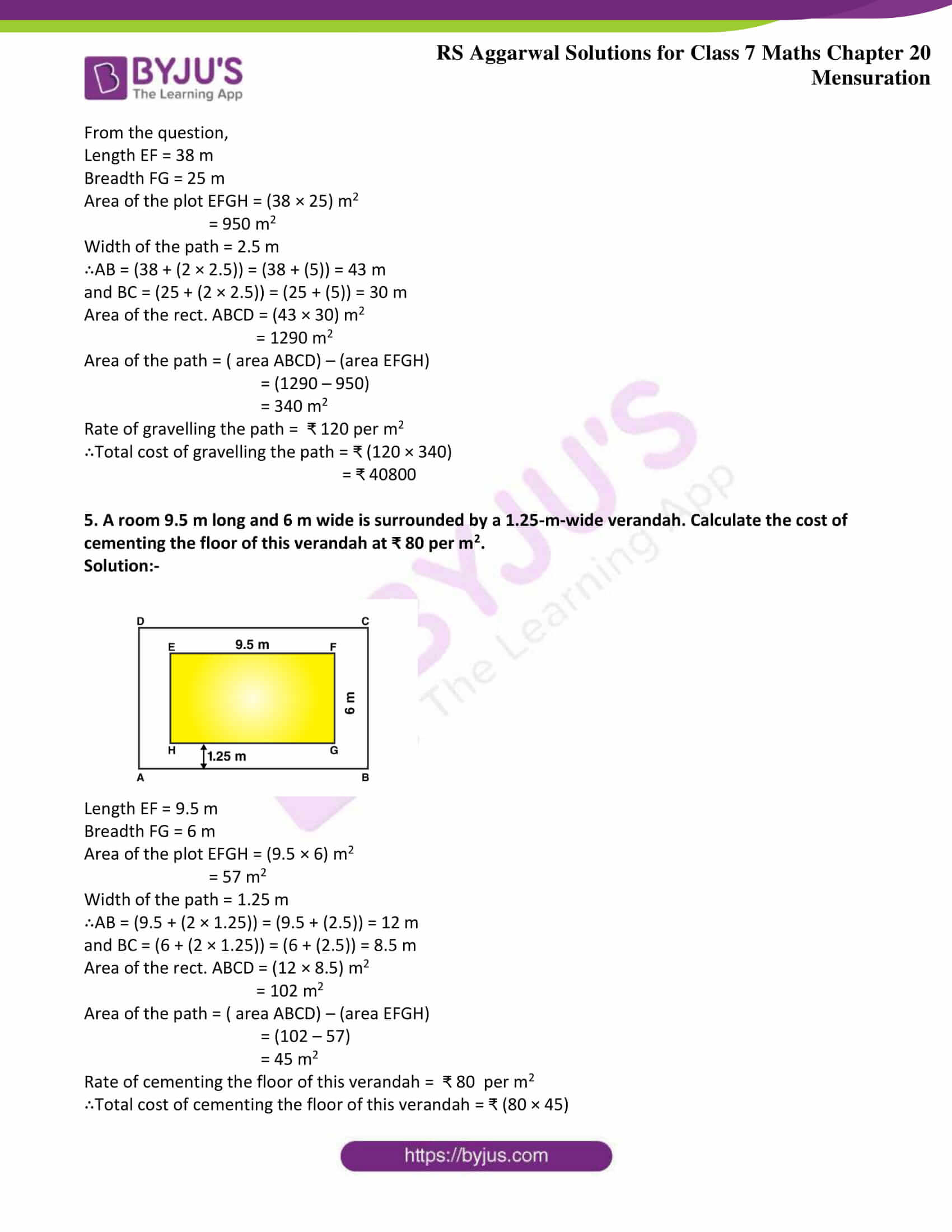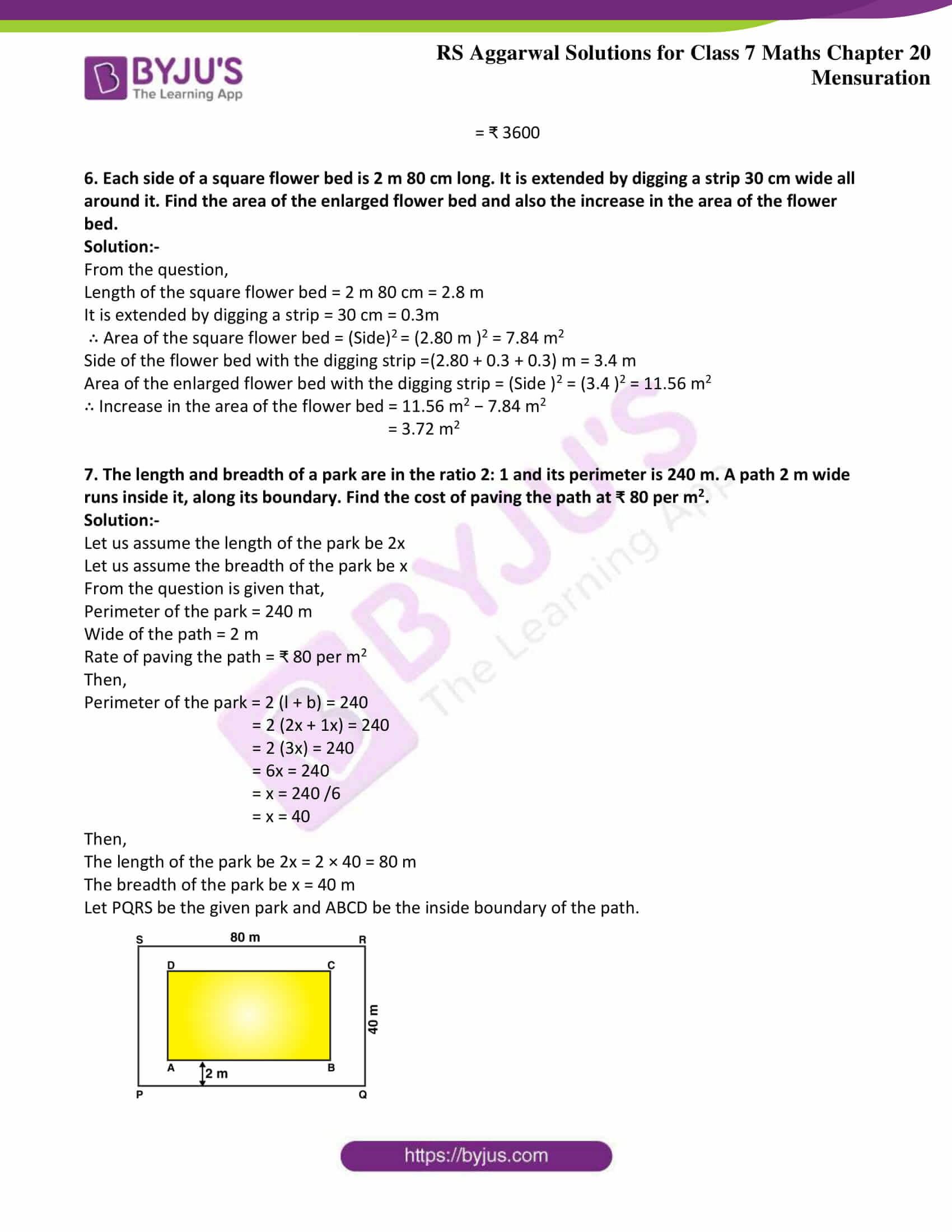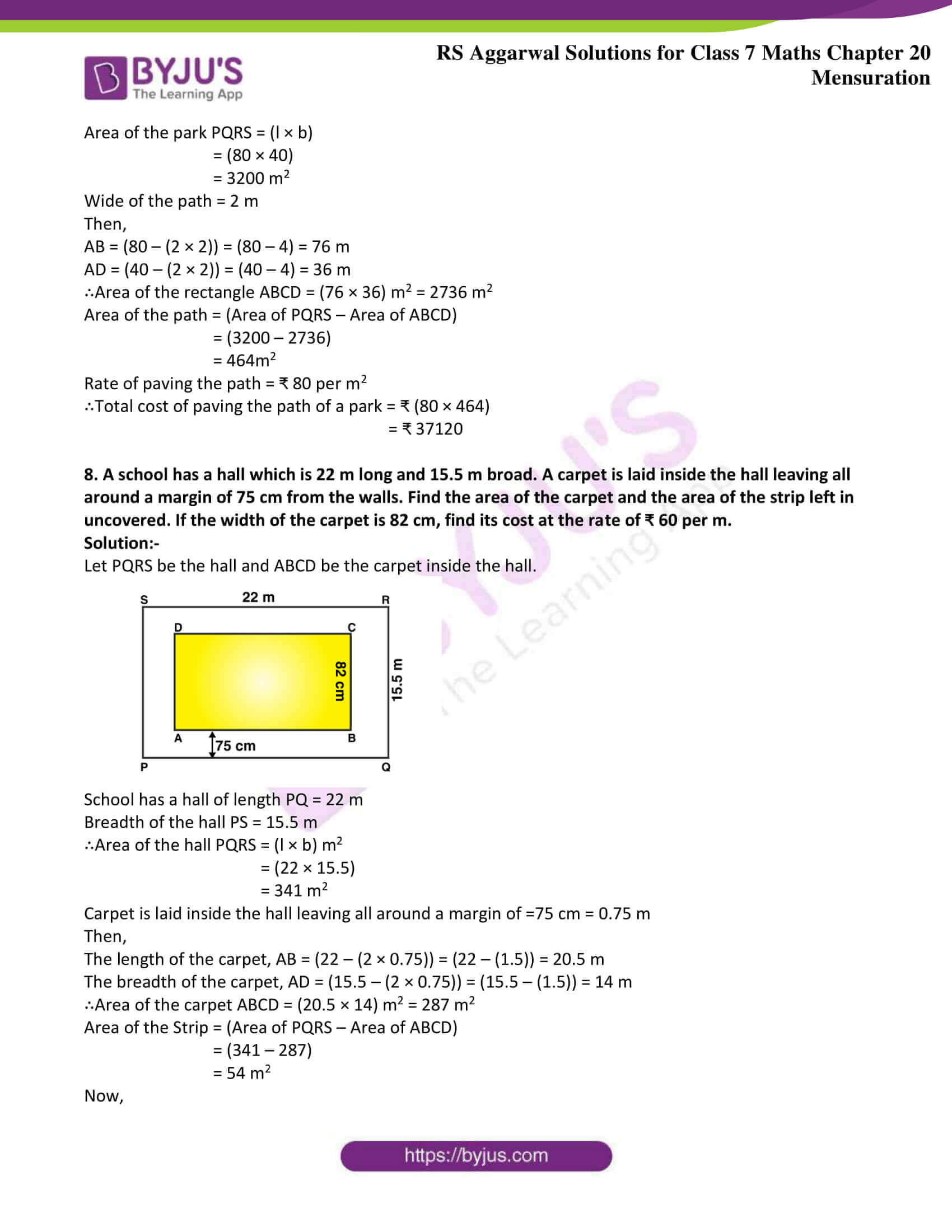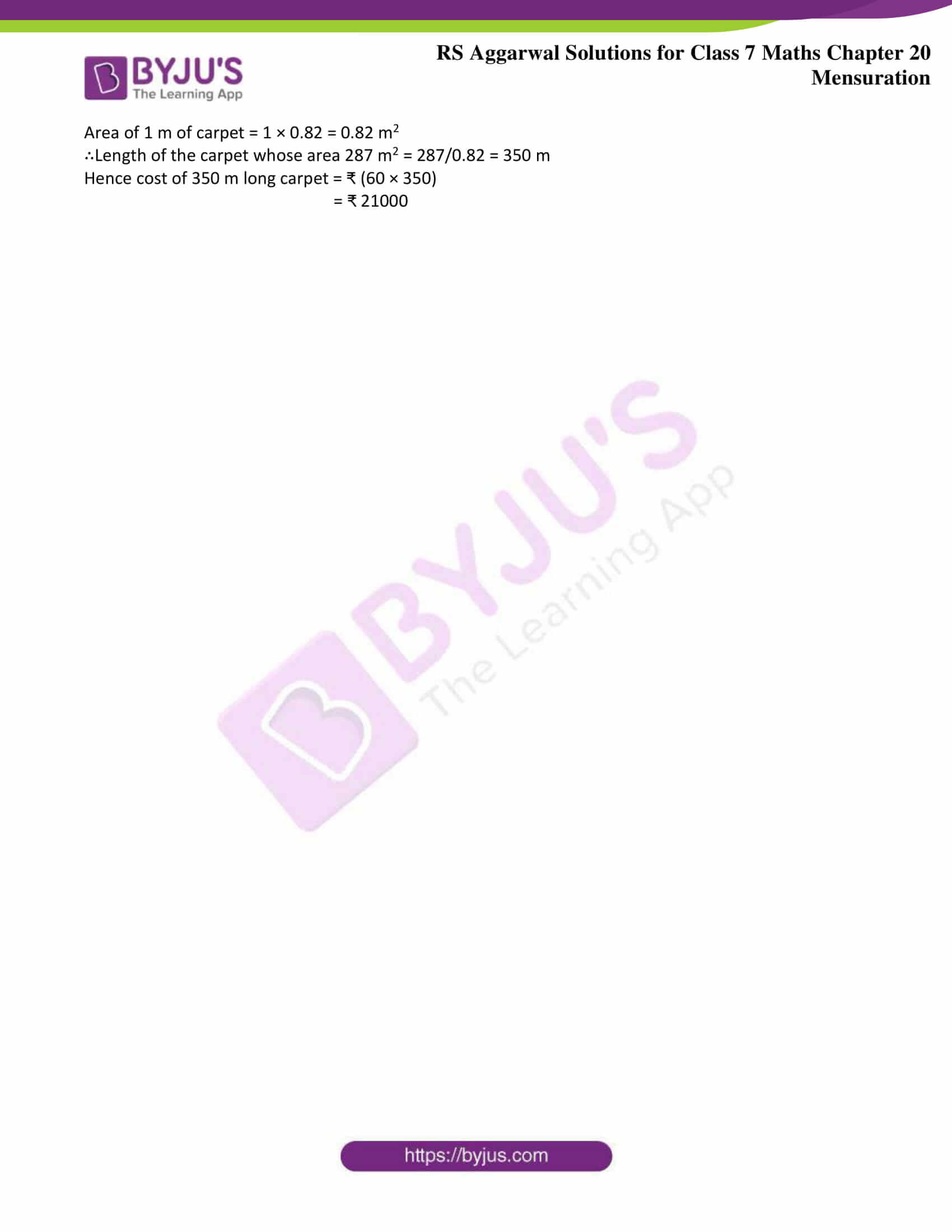### Access answers to Maths RS Aggarwal Solutions for Class 7 Chapter 20 – Mensuration Exercise 20B

1. A rectangular grassy plot is 75 m long and 60 m broad. It has a path of width 2 m all around it on the inside. Find the area of the path and the cost of constructing it at ₹ 125 per m2.

Solution:-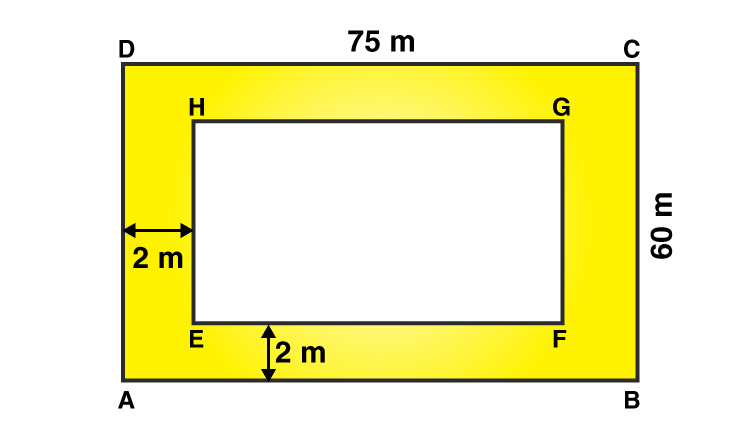Let ABCD be the given grassy plot and let EFGH be the inside boundary of the path,

Then,

Length AB = 75 m

Area of the plot ABCD = (75 × 60) m2

= 4500 m2

Width of the path = 2 m

∴EF = (75 – (2 × 2)) = (75 – (4)) =71 m

and FG = (60 – (2 × 2)) = (60 – (4)) = 56 m

Area of the rect. EFGH = (71 × 56) m2 = 3976 m2

Area of the gravel path = ( area ABCD) – (area EFGH)

= (4500 – 3976)

= 524 m2

Hence, cost of constructing the path = ₹ (524 × 125)

= ₹ 65500

2. A rectangle plot of land measures 95 m by 72 m. Inside the plot, a path of uniform width of 3.5 m is to be constructed all around. He rest of of the plot is to be laid with grass. Find the total expenses involved in constructing the path at ₹ 80per m2 and laying the grass at ₹ 40 per m2.

Solution:-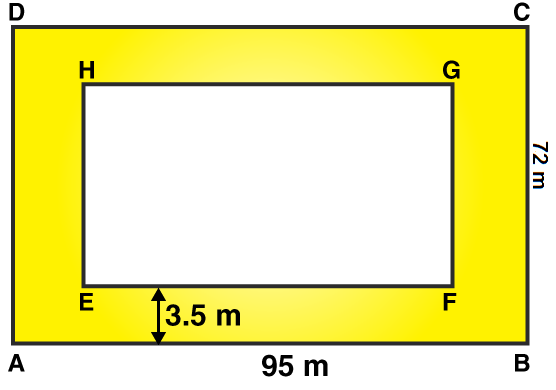Let ABCD be the given grassy plot and let EFGH be the inside boundary of the path,

Then,

Length AB = 95 m

Area of the plot ABCD = (95 × 72) m2

= 6840 m2

Width of the path = 3.5 m

∴EF = (95 – (2 × 3.5)) = (95 – (7)) =88 m

and FG = (72 – (2 × 3.5)) = (72 – (7)) = 65 m

Area of the rect. EFGH = (88 × 65) m2

= 5720 m2

Area of the gravel path = ( area ABCD) – (area EFGH)

= (6840 – 5720)

= 1120 m2

Rate of constructing the path = ₹ 80 per m2

∴Total cost of constructing the path = ₹ (1120 × 80)

= ₹ 89600

Rate of laying the grass on the plot EFGH = ₹ 40 per m2

∴Total cost of laying the grass on the plot = ₹ (5720 × 40)

= ₹ 228800

∴ the total expenses involved in constructing the path = ₹ (89600 + 228800)

= ₹ 318400

3. A saree is 5 m long and 1.3 m wide. A border of width 25 cm is printed along its sides. Find the cost of printing the border at ₹ 1 per 10 cm2.

Solution:-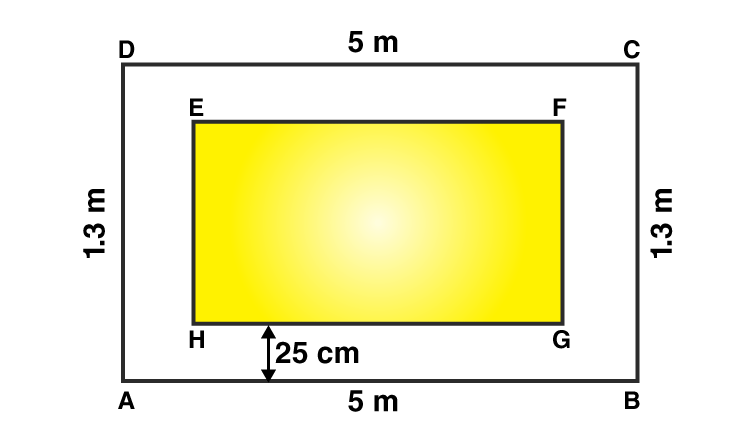Let ABCD be the saree and let EFGH be the part without border,

Then,

Length AB = 5 m

Area of the plot ABCD = (5 × 1.3) m2

= 6.5 m2

Width of the path = 25 cm = (25/100) = 0.25 m

∴EF = (5 – (2 × 0.25)) = (5 – (0.5)) =4.5 m

and FG = (1.3 – (2 × 0.25)) = (1.3 – (0.5)) = 0.8 m

Area of the rect. EFGH = (4.5 × 0.8) m2

= 3.6 m2

Area of the border = ( area ABCD) – (area EFGH)

= (6.5 – 3.6)

= 2.9 m2

= 2.9 × 1000

= 29000 cm2

Rate of printing the Border = ₹ 1 per 10 cm2

∴Total cost of printing the border = ₹ (1 × 29000)/10

= ₹ 2900

4. A rectangular grassy lawn measuring 38 m by 25 m has been surrounded externally by a 2.5-m-wide path. Calculate the cost of gravelling the path at the rate of ₹ 120 per m2.

Solution:-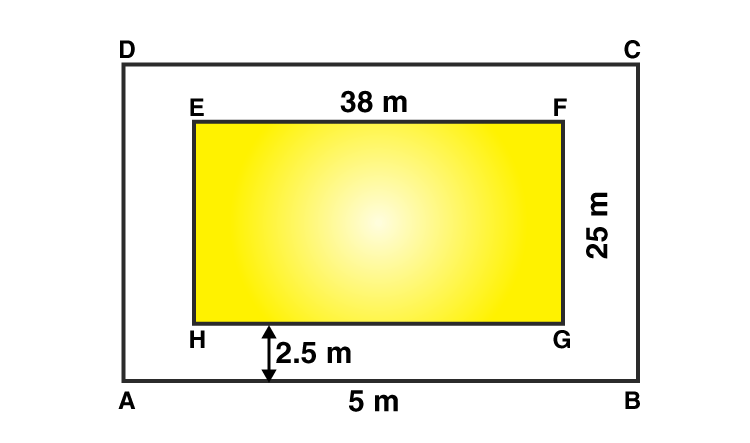From the question,

Length EF = 38 m

Area of the plot EFGH = (38 × 25) m2

= 950 m2

Width of the path = 2.5 m

∴AB = (38 + (2 × 2.5)) = (38 + (5)) = 43 m

and BC = (25 + (2 × 2.5)) = (25 + (5)) = 30 m

Area of the rect. ABCD = (43 × 30) m2

= 1290 m2

Area of the path = ( area ABCD) – (area EFGH)

= (1290 – 950)

= 340 m2

Rate of gravelling the path = ₹ 120 per m2

∴Total cost of gravelling the path = ₹ (120 × 340)

= ₹ 40800

5. A room 9.5 m long and 6 m wide is surrounded by a 1.25-m-wide verandah. Calculate the cost of cementing the floor of this verandah at ₹ 80 per m2.

Solution:-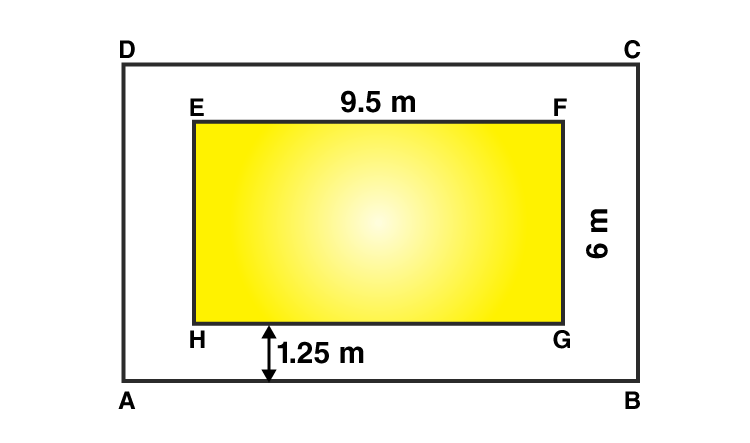Length EF = 9.5 m

Area of the plot EFGH = (9.5 × 6) m2

= 57 m2

Width of the path = 1.25 m

∴AB = (9.5 + (2 × 1.25)) = (9.5 + (2.5)) = 12 m

and BC = (6 + (2 × 1.25)) = (6 + (2.5)) = 8.5 m

Area of the rect. ABCD = (12 × 8.5) m2

= 102 m2

Area of the path = ( area ABCD) – (area EFGH)

= (102 – 57)

= 45 m2

Rate of cementing the floor of this verandah = ₹ 80 per m2

∴Total cost of cementing the floor of this verandah = ₹ (80 × 45)

= ₹ 3600

6. Each side of a square flower bed is 2 m 80 cm long. It is extended by digging a strip 30 cm wide all around it. Find the area of the enlarged flower bed and also the increase in the area of the flower bed.

Solution:-

From the question,

Length of the square flower bed = 2 m 80 cm = 2.8 m

It is extended by digging a strip = 30 cm = 0.3m

∴ Area of the square flower bed = (Side)= (2.80 m )2 = 7.84 m2

Side of the flower bed with the digging strip =(2.80 + 0.3 + 0.3) m = 3.4 m

Area of the enlarged flower bed with the digging strip = (Side )2 = (3.4 )2 = 11.56 m2

∴ Increase in the area of the flower bed = 11.56 m2 − 7.84 m2

= 3.72 m2

7. The length and breadth of a park are in the ratio 2: 1 and its perimeter is 240 m. A path 2 m wide runs inside it, along its boundary. Find the cost of paving the path at ₹ 80 per m2.

Solution:-

Let us assume the length of the park be 2x

Let us assume the breadth of the park be x

From the question is given that,

Perimeter of the park = 240 m

Wide of the path = 2 m

Rate of paving the path = ₹ 80 per m2

Then,

Perimeter of the park = 2 (l + b) = 240

= 2 (2x + 1x) = 240

= 2 (3x) = 240

= 6x = 240

= x = 240 /6

= x = 40

Then,

The length of the park be 2x = 2 × 40 = 80 m

The breadth of the park be x = 40 m

Let PQRS be the given park and ABCD be the inside boundary of the path.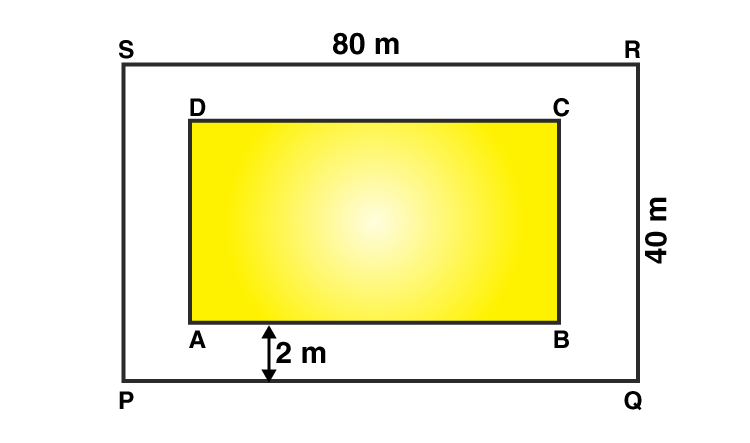Area of the park PQRS = (l × b)

= (80 × 40)

= 3200 m2

Wide of the path = 2 m

Then,

AB = (80 – (2 × 2)) = (80 – 4) = 76 m

AD = (40 – (2 × 2)) = (40 – 4) = 36 m

∴Area of the rectangle ABCD = (76 × 36) m2 = 2736 m2

Area of the path = (Area of PQRS – Area of ABCD)

= (3200 – 2736)

= 464m2

Rate of paving the path = ₹ 80 per m2

∴Total cost of paving the path of a park = ₹ (80 × 464)

= ₹ 37120

8. A school has a hall which is 22 m long and 15.5 m broad. A carpet is laid inside the hall leaving all around a margin of 75 cm from the walls. Find the area of the carpet and the area of the strip left in uncovered. If the width of the carpet is 82 cm, find its cost at the rate of ₹ 60 per m.

Solution:-

Let PQRS be the hall and ABCD be the carpet inside the hall.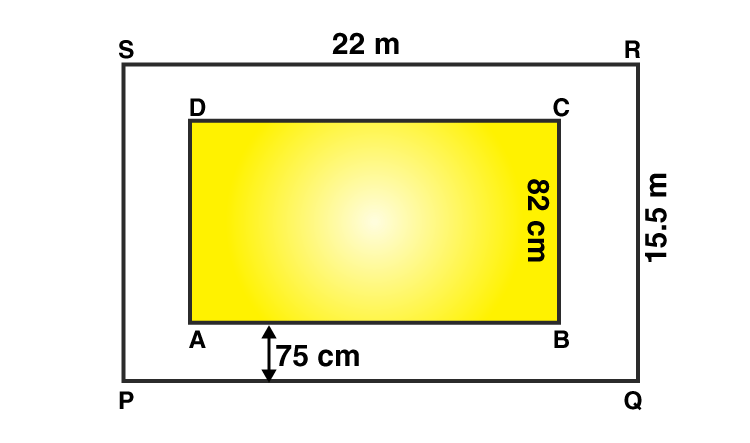School has a hall of length PQ = 22 m

Breadth of the hall PS = 15.5 m

∴Area of the hall PQRS = (l × b) m2

= (22 × 15.5)

= 341 m2

Carpet is laid inside the hall leaving all around a margin of =75 cm = 0.75 m

Then,

The length of the carpet, AB = (22 – (2 × 0.75)) = (22 – (1.5)) = 20.5 m

The breadth of the carpet, AD = (15.5 – (2 × 0.75)) = (15.5 – (1.5)) = 14 m

∴Area of the carpet ABCD = (20.5 × 14) m2 = 287 m2

Area of the Strip = (Area of PQRS – Area of ABCD)

= (341 – 287)

= 54 m2

Now,

Area of 1 m of carpet = 1 × 0.82 = 0.82 m2

∴Length of the carpet whose area 287 m2 = 287/0.82 = 350 m

Hence cost of 350 m long carpet = ₹ (60 × 350)

= ₹ 21000

### Access other exercises of RS Aggarwal Solutions For Class 7 Chapter 20 – Mensuration

Exercise 20A Solutions

Exercise 20C Solutions

Exercise 20D Solutions

Exercise 20E Solutions

Exercise 20F Solutions

Exercise 20G Solutions